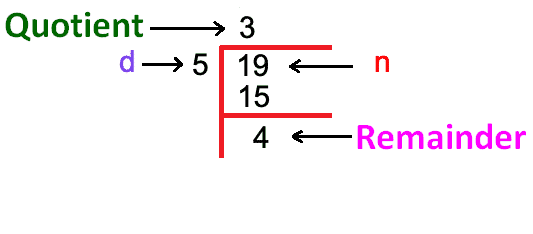# Remainder Calculator

Enter dividend and divisor in the below input fields. Hit the Calculate button to find the remainder using quotient and remainder calculator.Remainder calculator is used to find the remainder in a division operation. It is an online tool that carry out process of division with remainders.

In other words, it divides two numbers and gives the answer in integer instead of decimal value.

## What is a remainder?

Remainder can be defined as,

Remainder is a number that is left over after the division of two numbers. We get a remainder when a dividend is not completely divided by a divisor or when we get a quotient in decimal form.### Relationship of dividend, divisor, quotient, and remainder

The following equation shows how these four terms are related to each other.

d/n = q + r/n

Where,

d = number that divides (divisor),

n = number that gets divided (dividend),

q = result of division (quotient),

r = remainder.

Modulo operation also generates remainder when a dividend is divided by a divisor.

## How to calculate remainder?

To find the remainder in a division process, follow the below example.

Example:

Let’s divide 725 with 35.

Solution:

Divisor = 35

Dividend = 725

Step 1: Divide 725 with 35 using a calculator.

725/35 = 20.7143

Step 2: Separate the decimal value and whole number. Multiply the decimal value with the divisor.

20.71 = 20 and 0.7143

= 0.7143 × 35

= 25

25 is the remainder when we divide 725 with 35.

1. Remainder Definition (Illustrated Mathematics Dictionary) by mathsisfun.com